Action Research: The Model Method in Solving Word Problems – Connect Charter School

# Action Research: The Model Method in Solving Word Problems

-by Kevin Sonico

“Show me your ‘thinking’.”  “Explain your process.”  These are two statements that are often used to encourage students to communicate their strategies in solving a word problem.  In my experience, what is often produced, written, and described are algorithms, equations, and other symbolic representations.  Rarely have students submitted strategies that comprised of visual methods.  Because of this, I set out to see if there is an effect of explicitly teaching a visual strategy, namely the model method, in helping students solve problems.  Through the use of models, students worked on word problems around the concepts of fractions, decimals, percent, and ratios.  Described here are three examples of the many problems posed in class.

In addition to helping students solve word problems through the use of models, I hoped to equip students with a tool that will help them develop their visualization skills. “Students are expected to develop visualization skills to assist in processing information, making connections, and solving problems.” (The Alberta K-9 Mathematics Program of Studies with Achievement Indicators, 2007)  The curriculum describes visualization as one of the “critical components that students must encounter in a mathematics program in order to achieve the goals of mathematics education and embrace lifelong learning in mathematics.”  Furthermore, this document explicitly outlines four specific outcomes of the Math 8 curriculum that expect students to exhibit their understanding in concrete, pictorial, and symbolic ways (Appendix A).

The model method is sometimes referred to as the Singapore bar model method as students in Singapore are taught how to use bar models in solving word problems.  The benefits of teaching this visual strategy have been noted. Hoven and Garelick (2007) stated that “[i]n Singapore, where 4th and 8th grade students consistently come in first on international math exams, students learn how to solve problems using the bar model technique.”   Hong, K.T., et al. (2009) wrote, “through the construction of a pictorial model…, students gain better understanding of the problem and develop their abilities in mathematical thinking and problem solving.”

The Transportation Problem

The bar model method is systematic and formulaic; it provides a framework for students to follow when solving word problems. Students draw bars (hence the name) to represent an unknown quantity.  They then divide the bars into parts.  Within and around the bars, students write labels and other information from the given problem.  The set-up of the bars and the labels act as a “picture” of how students visualize the numbers and the relationship between and among the given information.  The Transportation problem was one of the first problems that students solved after having been introduced to the model method.  (Challenging Word Problems, 2011)

One-third of the students go to school by bus.  One-fourth of the remaining students go to school by car.  The rest of them either ride a bike or walk to school.  If 90 students go to school by car, how many students are there in the school?

To illustrate how the model method works, student exemplars (Figure 1) from the Transportation problem are included below.

Figure 1 A, B, C, and D: Variations of the bar model method.  A and B: Both students used similar labeling techniques.  C: Student shows intricate knowledge of equivalent fractions and visual division of fractions.  D: Student depicts the use of a number line which is similar to the bar models shown in Figures 1A and B.

C.

D.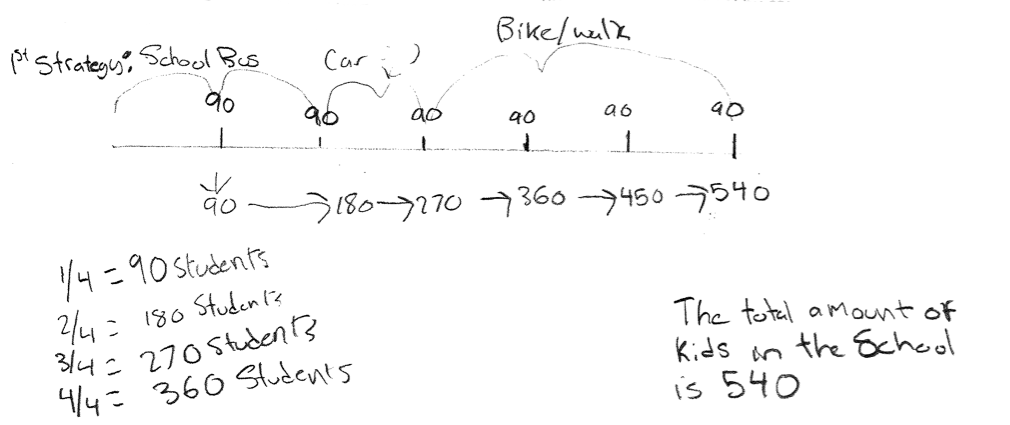Although it is a set of systematic procedures, students created varying forms of the models to illustrate their understanding of the problem (Figure 1).  Based on a survey of all of the strategies employed by students, over half of them chose to solve it in a symbolic manner (Figure 2), that is through the use of numbers, symbols, and algorithms (Figure 3).  The second most common strategy was the use models.  Interestingly, when students were asked to use a verification strategy, that is, to use another tool to check their solutions, the model method was the most popular, followed by symbolic strategies.

Figure 2: A breakdown of the main and verification strategies used by students in solving the Transportation problem.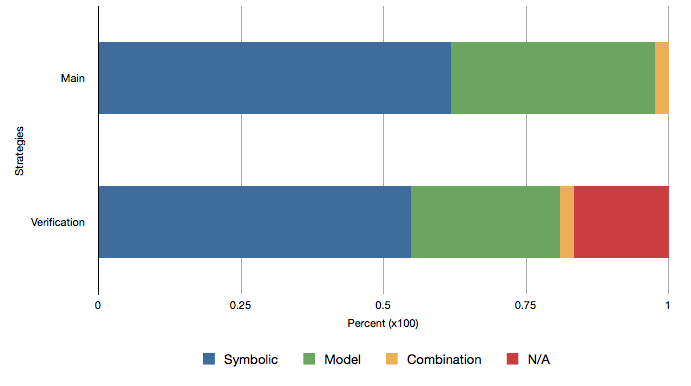Figure 3: Two examples of a symbolic way of solving of the Transportation problem.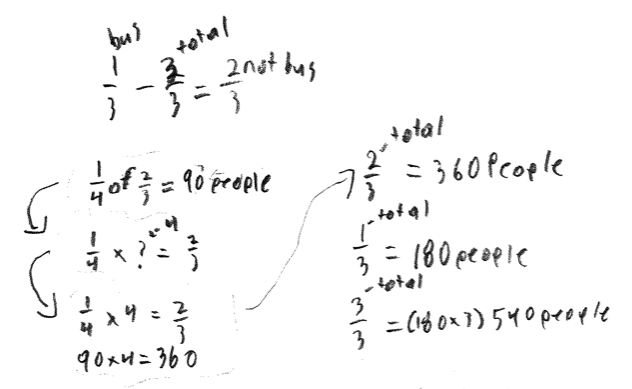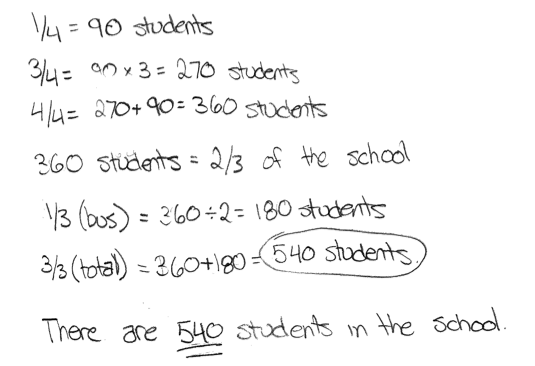After having been introduced to the model method, I expected most students to utilise this strategy in their solution.  The results, however, indicated otherwise; most students solved it through symbolic means.  This is perhaps due to students’ familiarity and comfort in using symbols and algorithms.  When performing a task with a tool, most of us will probably reach for the one with which we are most familiar.  We have a preferred way of doing things. This is in no way different from the results of the survey. Students have a preferred method of solving – not necessarily all word problems, but this word problem in particular.  The overall survey results also point to the explanation that even though a new skill or strategy has been introduced, it does not necessarily mean that students will readily employ it or easily adopt it in their practice.  This leads me to wonder: if students were taught the model method in earlier grades, how familiar would they be by Grade 8 and how frequently would they choose it to solve word problems?  Conversely, if students were not exposed to the model method at all, what other tools or strategies would they have employed to verify their solutions?  Although a small portion of students were unable to come up with an alternate strategy, most students provided a verification strategy that utilised the model method.  With increased exposure to, time with, and practice in using the model method, I expect students to become even more comfortable with it.

The Pet Problem

We typically hold a “congress” after solving word problems.  As described by Fosnot & Dolk. (2002), a math congress allows students to share, discuss, and defend individual strategies.  Through rich discussions, students can pull common threads among the many methods, and can start to appreciate the interconnectedness among the concepts and different strategies.  For the Transportation problem, however, I opted not to conduct the congress. I wanted to see how the students will solve a modified version of the problem, called the Pet problem, after more months of exposure in using models.  I also wanted to see if there was an effect or a shift in the number of students who will use the model method as a main strategy.  Sharing our strategies through the congress would have also influenced their strategies for the Pet problem.   The Pet problem read:

One-third of the people surveyed prefer cats as pets.  One-fourth of the remaining participants of the survey preferred fish.  The rest of the people surveyed preferred dogs.  If 12 people preferred fish, how many people in total were surveyed?

Figure 4: A breakdown of the main and verification strategies used by students in solving the

Pet problem.After months of using the model method, the results were as I expected them to be (Figure 4) .  It indicated that most students used the model method as their main strategy and some form of symbolic method as a verification strategy. This result was the opposite of the results for the earlier Transportation problem, where most students solved it through symbolic means.  Students perhaps became more comfortable in using the model method.  Perhaps some saw the merit in using and drawing bars over using an algorithm.  Or perhaps they found it a better fitting tool for the problem.  A student reflected that using models was “easier for [him] to process the question”, and it “helped visualize the problem”.  In one instance, a student who used the model method to verify his answer saw that his original solution was incorrect.  He wrote, “I think my algorithm was inaccurate because I couldn’t model what the question asks” (Figure 7).  In word problems like the Pet or Transportation problem, the abundance of numerical information for students to consider can be overwhelming or confusing.  The strength of using the bar model is in its flexibility and its ability to allow for students to visually organize the information in a way that may make more sense to them.

Figure 5: Two examples of students’ solution to the Pet problem using the model method.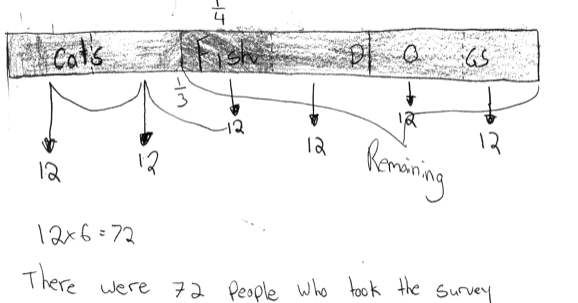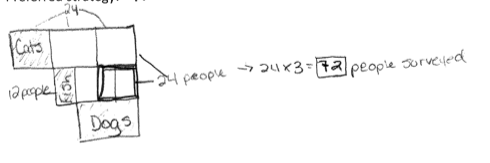After having given the two problems to students, I also wanted to compare the proportion of students who answered the problems correctly.  Months earlier, I was not pleased with the percentage of students who answered the Transportation problem incorrectly (Figure 8).  About half of them committed errors like using equations and algorithms that were not mathematically logical.  In contrast, most students answered the Pet problem correctly. Although a strong, positive correlation between the use of bar models and more accurate answers cannot be made, it is worth suggesting that perhaps it is due to students’ increased confidence in using the bar models that have allowed them to better understand the problem.  It is also possible that they have improved their grasp of fraction concepts .  For whatever probable reasons there are for this shift, students were readily reaching for the model method as a tool.  They saw it as a good fit with the word problem.  This is noteworthy because the Pet problem is fundamentally identical to the earlier Transportation problem, which was mainly solved symbolically.

Figure 6: A verification strategy which employed the multiplication of fractions algorithm.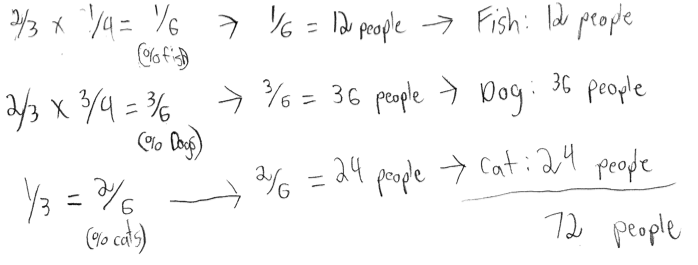Figure 7: Two strategies used by a student, where the model method was used in verifying the initial solution to the Pet problem. The student reflected how his preferred strategy (through symbolic method) was incorrect.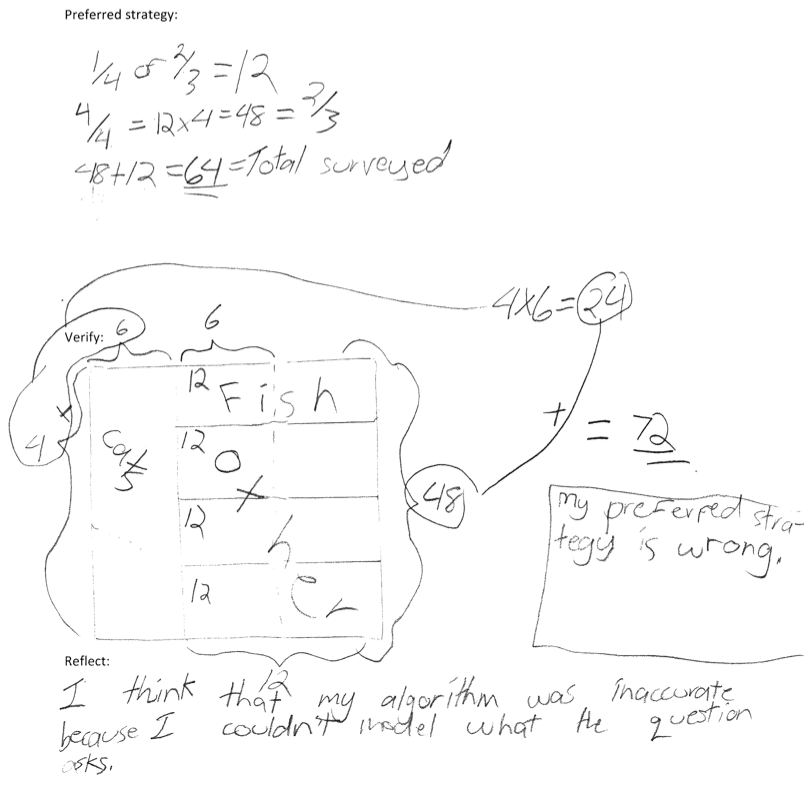Figure 8: Percentage of students who incorrectly answered the Transportation and Pet problems.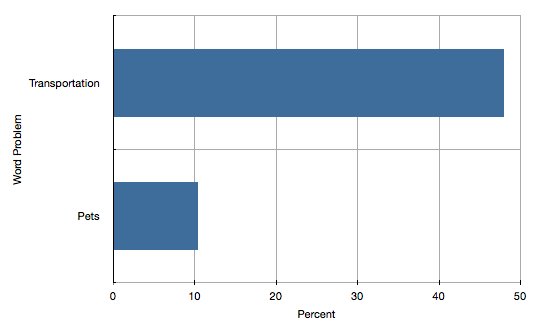The Interest Problem

The Transportation and Pet problems were similar in wording and in the relationships among the numbers. Both problems also contained data that students expected to “plug” into some formula or to use in their modeling.  The Interest problem, on the other hand, is different in that it is less verbose.  Taken from Nrich.Maths.org, the Interest problem asked, “If a sum that is invested gains 10% each year, how long will it be before it has doubled its value?”  Posing this word problem encouraged students to make conjectures and “tinker” with numbers.  These mathematical skills and habits of mind make the Interest problem a lot richer and more challenging in my opinion.  Because the question did not have the degree of detail or data like the Pet and Transportation problems, students who have relied heavily on given data may have found this a bit more challenging.  A student commented that this word problem was “more difficult because [he] didn’t have a total number to work with.”  Most students, however, managed to choose a number, such as \$1, \$10, or \$100, as a figure to use in their calculations and solutions.

Figure 9: A breakdown of the strategies used by students in solving the Interest problem.

According to the survey of student work, the overwhelming majority of students (approximately 90%) utilised symbolic methods while the rest used models to solve it (Figure 9).  Students probably chose to solve it symbollically as they saw the ease and efficiency of using numbers and symbols over drawing models for this particular problem.  “Calculating with number sense means that one should look at the numbers first and then decide on a strategy that is fitting – and efficient.” ( Fosnot et al., 2007)  A student commented that “using the unit value and a formula worked the best with [the Interest] problem; [He] wasn’t sure how [he] would make a visual representation.”  Another student reflected on the restriction of the model method when working with decimals.  This is an interesting comment because decimals are essentially fractions.  They both signify parts of a whole.  Even though the model method was not used by most, a student wrote, “I like visuals because they make me write out what I know.  I may not use a complete visual method but it helps me with stating what I know without rewriting the question.”

Although most students chose to solve the Interest problem symbolically, there was not one single algorithm or formula that was utilised by all (Figure 10).  Some students preferred to calculate the “unit value”, that is, the 1% value of their sum, then multiplied that by 10 to determine the 10% value (Figure 11A).  Others chose to divide the sum by 10 rather than 1, as they saw that 10 is a factor of 100, thereby bypassing the multiplication step performed when calculating the “unit value” (Figure 10B).  Then there were others who multiplied their sum by 1.1 to signify 110% growth (Figure 10C).  Regardless of the chosen calculation method, it is evident that students did not see the benefit of using models for this problem. An argument, however, can be made that using the model method may have influenced students to represent their understanding symbolically (Hoven et al., 2007).  Students may have made the leap from visual to symbolic thinking.  For instance, finding the unit value – a strategy that was used symbolically – is a hallmark step used in the model method. In using bars to represent units, students would calculate the value of a bar by dividing a sum by the number of bars.  In essence, that is similar to finding the unit value.

Figure 10: A breakdown of the symbolic strategies employed by students in solving the Interest problem.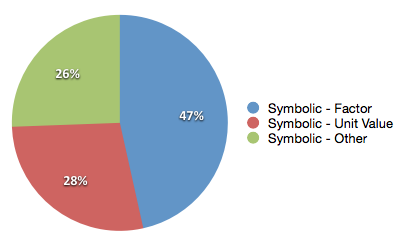Figure 11: Exemplars of students’ symbolic strategies in solving the Interest problem. A: student finding the “unit value” then multiplying by 10 to get 10% of sum; B: student using 10% by dividing it by 10; C: student multiplies sum by 1.1 to represent 110% growth.  D: model method used to demonstrate student’s understanding of the problem.

A.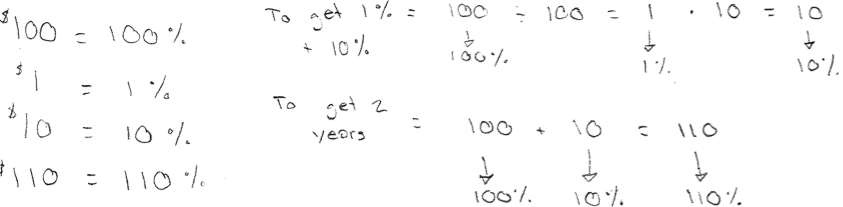B.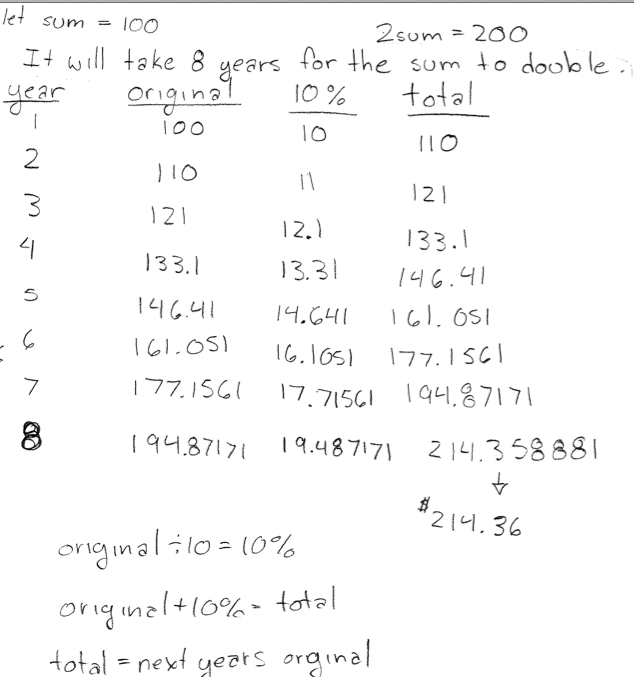C.

D.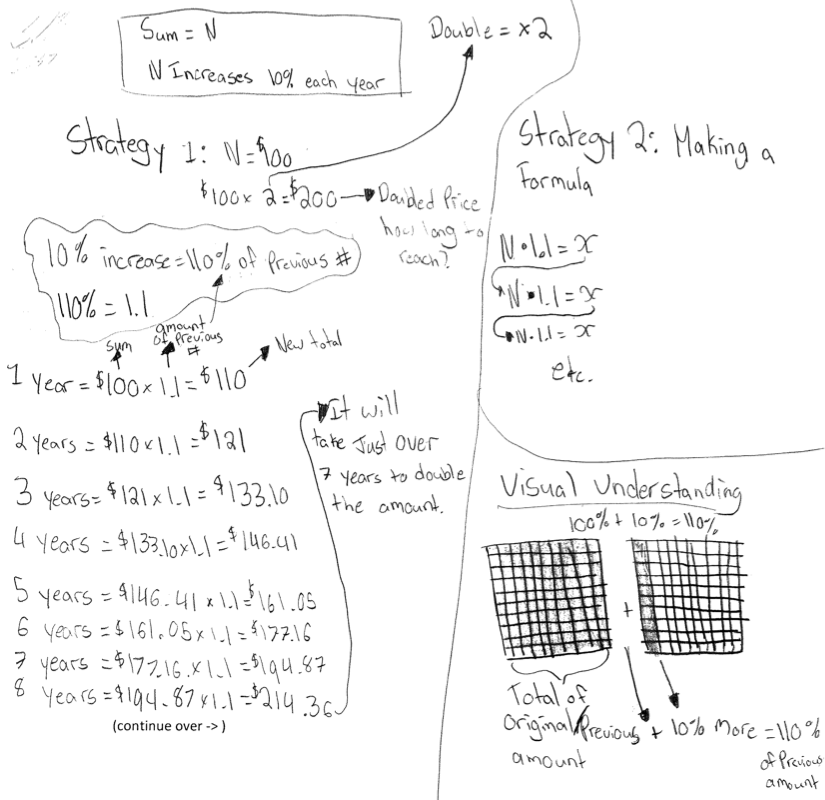Verifying

When we urge students to “check” their work, we expect them to critically look at their processes and evaluate the effectiveness of their methods and the accuracy of their work.  We do not expect them to passively read through their solutions.   Some students, however, may go through the verification process by simply doing that.  “Looking for errors by repeating exactly what has been done is a poor way to check.” (Mason, Burton, & Stacey, 1985).  In addition, students sometimes verify their solutions by working backwards.  This is not the most effective way to verify an answer to a word problem as students are merely performing the inverse operations of their original algorithms.  When the same data (including the solution) is entered into the inverse algorithm, one will mathematically arrive at the original number.  For example, if 1 + 1 = 2; then naturally 2 – 1 = 1.   This is a flawed form of verification as all it is checking for is the arithmetic – not the mathematical logic and numerical connections behind the data.

For some students, it can be a struggle to think of an alternate method of solving the problem.  When students are encouraged to provide another strategy, we are challenging them to see the problem through a different lens.  The model method might provide them with a different way of viewing and thinking through a word problem.  Before being introduced to drawing bars and using models, some might not even have thought of using a visual strategy in solving and verifying their solutions.  One student, who used a symbolic method as his first strategy to solve the Transportation problem and the model method as the second strategy, wrote,

I find the 2nd strategy easier, but if you know what your [sic] doing, the first is faster.  Strategy 1 is good because it is fast and efficient but the 2nd is good to check your work.

This sums up a common sentiment among students who have grasped the abstract nature of numbers and have decided on an efficient algorithm to solve it.  The challenge with algorithms is that it is difficult to gauge whether or not the student has made sense of the numbers unless further questioning or probing is done.  With the model method, we can at least see how students interpret the data as they relate to each other.  For students who have a difficult time verbalizing or describing the intricate and complicated calculations that they performed mentally, the model method may also provide them with a way to communicate their complex calculations.

For students who struggled with coming up with their main strategy, in other words, those who are left in the “stuck” phase, they may have benefited from being introduced to the model method.  Although it is “natural” to be in the “stuck” phase (Mason et al., 1985),  using the model method might allow a student to answer the question “So now what?” after reading a word problem.  In the Pet problem, 8% of students, who used the model method as their main strategy, were unable to come up with a verification strategy.  Without using the model method, this 8% of students would have been left in the “stuck” phase and not have answered the problem altogether.

Conclusion

The model method can look formulaic and prescriptive, in that it is a generalized procedure, comprised of labeling bars and identifying the given data and missing information.  An argument can be made that this is no different from memorizing the algorithm to short or long division, for instance.  I do not disagree with the notion that it can be similar because of the procedural nature of the method.  However, what distinguishes the model method from other forms of algorithm is that it provides a framework that makes sense to students.  Students who have difficulty understanding the logic behind the steps of an algorithm may have an easier time understanding the practice of drawing bars. When reflecting on the model method, a student commented that it was “helpful in visualizing the questions and help[ed] [him] to understand why the formulas work not just how they work.”  (Appendix D: Reflections on Model Method)

Figure 12 Students’ responses to the question, “How was the model method helpful in solving and understand word problems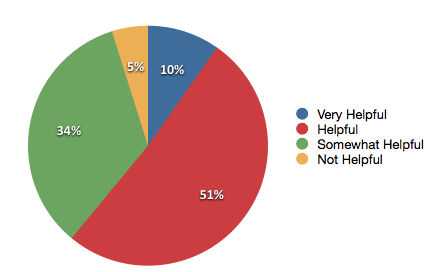When students were asked about how helpful the model method was in understanding and solving word problems, over half of the students found it either helpful or very helpful; while 33% of students found it only somewhat helpful, and 5% found it not helpful at all (Figure 11).  A student commented,
[t]he bar models helped me to visually represent numbers in my head.  They are a good method for checking you (sic) work or when you are stuck. It also provided proof that the algorithm method is correct.

Another student wrote,

Models can show you things a (sic) algorithm cannot.  A model can show you the magnitude, and can also visually prove to you if something is right or wrong.  You can look at it and say “hmm, that doesn’t look right”.  A model can also put things into perspective.  A (sic) algorithm gives you an answer.  A model SHOWS you the answer…

When students struggle with a word problem, the challenge at times is not knowing where to start.  Whether we have explicitly taught an algorithm or implicitly modeled a strategy in our practice, we have equipped students in the past with word problem solving strategies like underlining important texts or listing the “knowns”.  We have recited “think alouds” – questions that we want students to be asking themselves when thinking through word problems.  For one student, the model method helps to “un-jumble” her thoughts.  The benefit to using visual models is that it allows for students to start somewhere – to start drawing the situation, to start making sense of the numbers.  For others who are able to process the numbers through symbolic means, the model method provides them with a verification strategy. The true strength of the model may also lie in its ability to help students make that connection, a bridge, from concrete to abstract, and vice versa.

The intent of this action research was not to eradicate the use or minimize the power of symbolic representation. . “[The] beauty [of an algorithm] was that they were generalized procedures that could be used as efficient computat
ion strategies.” (Fosnot
et al., 2002)

Algorithms…do have an important place in mathematics.  After students have a deep understanding of number relationships and operations and have developed a repertoire of computation strategies, they may find it interesting to investigate why the traditional computation algorithms work

Therefore, the power of any symbolic representation including algorithms lies in students’ understanding of how it came about, its derivation. Equally powerful is when a student produces a formula or an algorithm that makes mathematical sense to solve a problem.

A common attitude that was prevalent during this action research was hesitation or resistance. Some students found it tedious and time-consuming to draw a pictorial representation of a concept that can easily be represented with symbols and numbers. “Why do we have to draw this if we can show it with numbers?” or “Why do we have to draw in Grade 8?” To this, I simply replied with an analogy.  Think of the model method as a pair of scissors. It is a tool for cutting things.  In the world of cutting tools, there are plenty of them: handsaw,  jigsaw,  bandsaw, paring knife, serrated knife, razor blade, etc.  The more tools one owns, the better the choices one has for the job.  Learning how to use models adds another tool that one can use.

Some may point out that the method of emphasizing the use of one visual strategy is contrary to the inquiry-based model or problem-based facet of our practice.  However, when the focus shifts to the importance placed on having the ability to represent a problem from different perspectives, it is accomplishing that facet of inquiry where students are encouraged to look at multiple points of view.

There are several possible extensions of this action research.  First, it might be of interest to see how students will use and adopt the model method in higher grades where there will be increasing demands to symbolically represent their thoughts.  Will they abandon the model method or will they use it to help them unpack the complexities of more advanced concepts?   A student commented,

the bar model method was very helpful in certain circumstances.  However, I was not able to use it in as many situations as I would have liked to.  Many times, I didn’t know how the bar model method would have fit into the question, and I just ended up doing the algorithm or doing it in my head.  I thought it was made for basic problems, not for some of the problems we had to do.  In later years, I will have more challenging problems in math, and I don’t believe I will be able to use the bar model method very often.

As it is closely tied to the model method, observing how students develop their algebraic reasoning would be interesting (Hoven et al., 2007).  Second, it might be noteworthy to see how earlier grades can incorporate the model method in their curricula.  The resistance and hesitation to drawing models in later years might be mitigated if/when we start/continue to encourage students to visually represent their “thinking”.  Third, the impact of technology in demonstrating the model method could be explored.  Although students have found it to be effective, some commented on the inefficiency and time-consuming nature of the drawing models.  Perhaps apps or online manipulative sites such as the National Library of Virtual Manipulatives could be developed and evaluated for efficiency and effectiveness in helping students develop their skills in solving word problems and in visualizing abstract concepts.

References
Alberta Education, The Alberta K-9 mathematics program of studies with achievement indicators (2007).  Alberta, Canada.

Challenging word problems (2011).  Singapore: Marshall Cavendish Education.

Fosnot, C. T., & Dolk, M. (2002). Young mathematicians at work, constructing fractions, decimals, and percents. Heinemann Educational Books.

Hoven, J. & Garelick, B. (2007). Singapore math: simple or complex?. Educational Leadership (vol. 65 no 3).

Kho, T. H., Yeo, S. M., & Lim, J. (2009). The Singapore model method for learning mathematics. Singapore: Panpac Education.

Mason, J., Burton, L., & Stacey, K. (1985).  Thinking mathematically.  Essex, UK: Addison-Wesley Publisher Limited.

Resources
Clark, A. (2010). Singapore math: a visual approach to word problems. Houghton Mifflin Harcourt.

Challenging word problems (2011).  Singapore: Marshall Cavendish Education.

Har, Y.B. Bar Modeling: A Problem-solving Tool; from Research to Practice.  Singapore: Marshall Cavendish Int Pte Ltd.

Kho, T. H., Yeo, S. M., & Lim, J. (2009). The Singapore model method for learning mathematics. Singapore: Panpac Education.

Appendix A

Specific outcomes that outline expectations for students to demonstrate their understanding of a concept concretely, pictorially, and symbolically.  Taken from the Math 8 Program of Studies, The Alberta K-9 Mathematics Program of Studies with Achievement Indicators (2007).

Strand: Number
It is expected that students will:

• Demonstrate an understanding of perfect squares and square roots, concretely, pictorially and symbolically (limited to whole numbers).
• Demonstrate an understanding of multiplying and dividing positive fractions and mixed numbers, concretely, pictorially and symbolically.
• Demonstrate an understanding of multiplication and division of integers, concretely, pictorially and symbolically.

Strand: Patterns and Relations
It is expected that students will:

• Model and solve problems, concretely, pictorially and symbolically, using linear equations…

Appendix B

Accuracy of Solutions to the Interest Problem

An interesting result from the Interest problem was that over half of the students answered the question incorrectly (Fig. 13).  And most of these errors came from assuming that the amount or value of 10% is consistent from year to year.  Because of this assumption, they predicted for the money to double in 10 years (Fig. 14).  Whether or not the model method could have prevented this error is unknown.  A source for this mistake may very well be in the wording of the problem.  Because it does not specify that 10% would be added to the previous year’s amount, most students who incorrectly answered it assumed that it would be added to the original amount (Fig. 14). In this way, their answers are accurate. The concept of simple interest and compound interest were not explored before this problem was introduced so the notion of earning “interest on interest” was probably not even considered by the students.

Figure 13: Percentage of correct and incorrect answers to the Interest problem, and percentage of students who made an error in assuming that 10% value year to year is consistent.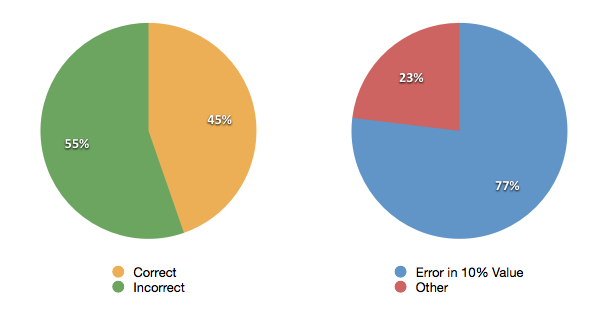Figure 14: Student misinterpretation of the Interest problem.  The majority of errors were committed with this assumption, that is, 10% was added to the original invested sum, rather than 10% to the previous year’s sum.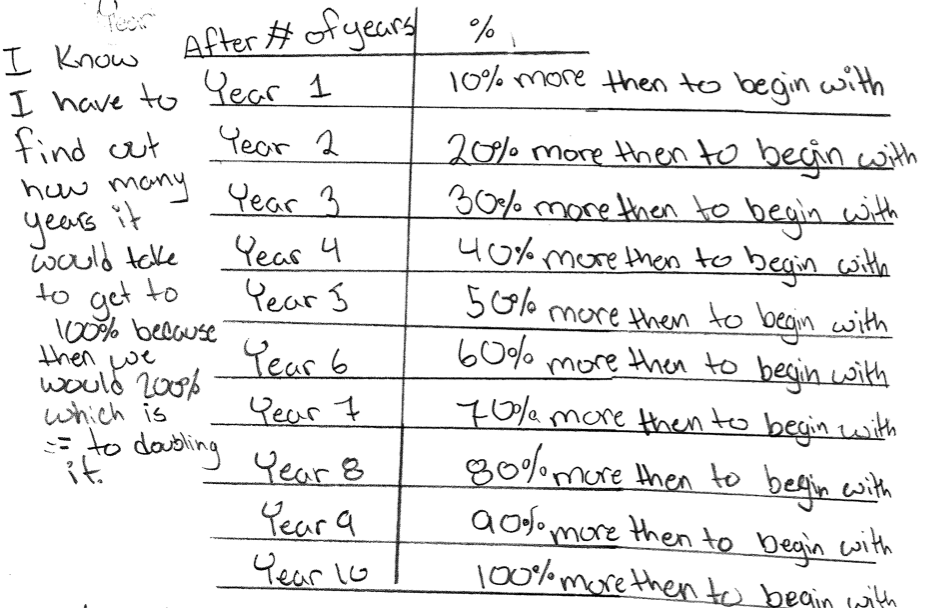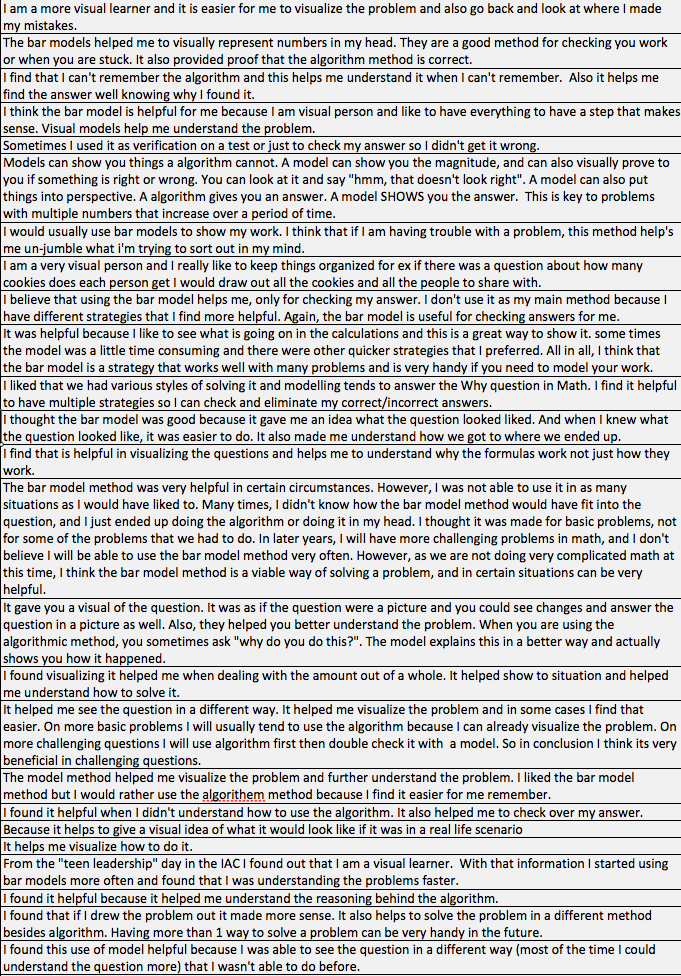Appendix D: Comments of students who saw little to no help in using the model method in solving word problems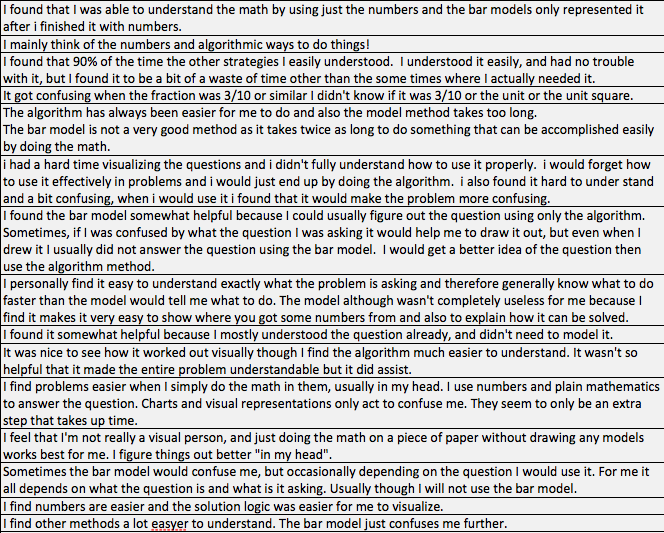1.Garry McKinnon says:
2.Garry McKinnon says: